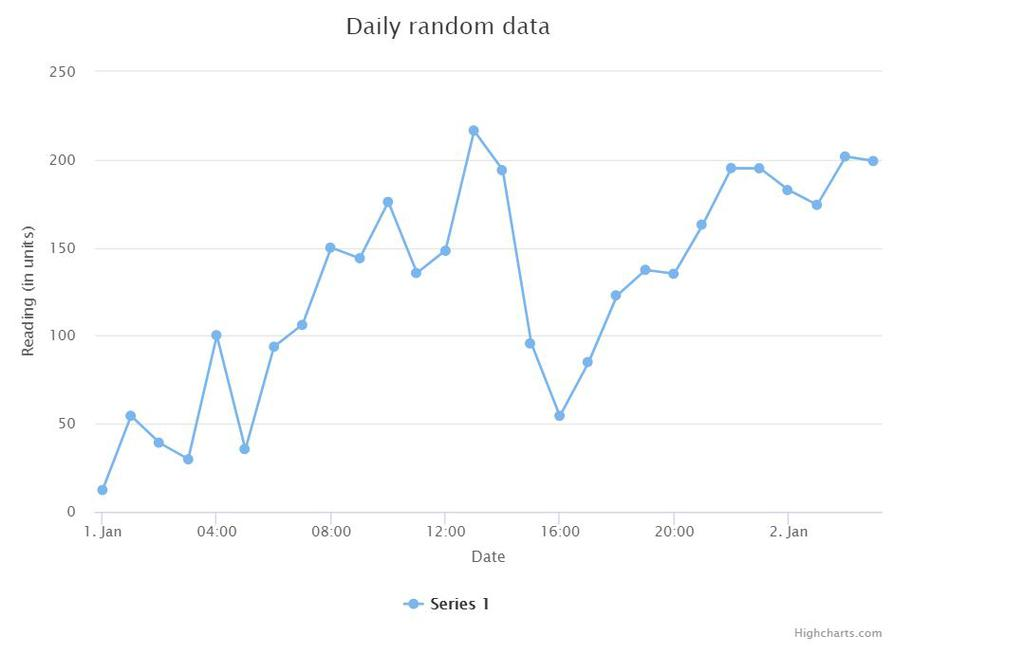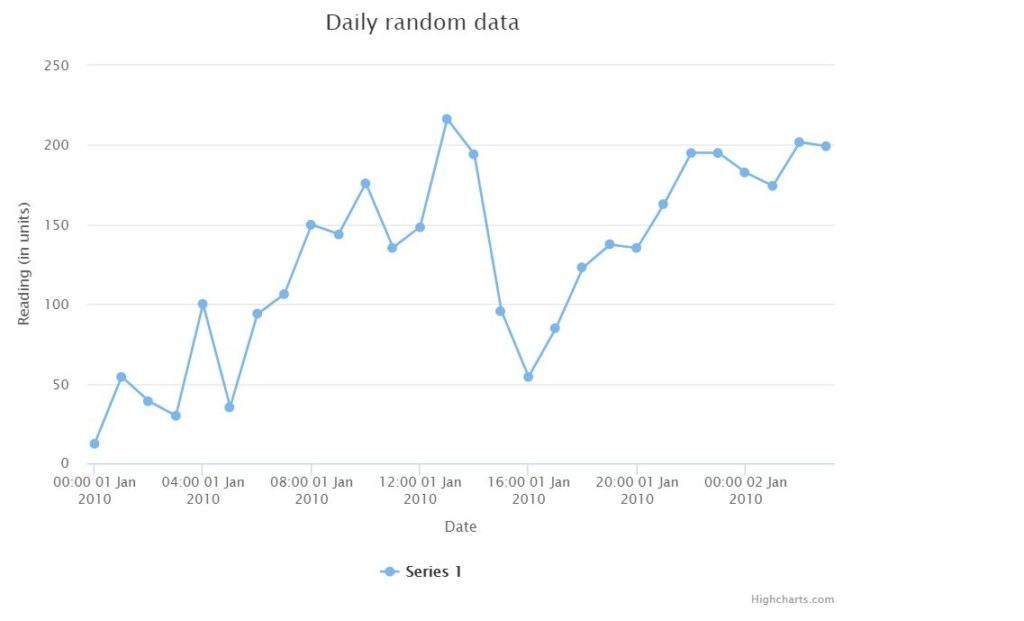# How to get highcharts dates in the x-axis ?

Graphs or charts are the best way to represent data as they are more user-friendly than viewing raw data. It also makes it easy to perform analytics on the data. There are many libraries in JavaScript that make it easy to create these visualizations and use them in mobile or web applications. One such library is Highcharts JS. It is a SVG based multi-platform JavaScript charting library that makes it easy to add interactive visualizations to applications.

Example: The below example shows a simple line chart with random data and the x-axis having DateTime type. The code below will be used to define the HTML document where the graph would be drawn.

## html

 ` ` `<``html``> ` `  ``<``head``> ` `  ``<``title``>Highcharts JS line chart ` `     `  `  ``` `  ``<``script`  `    ``src``=``"https://code.highcharts.com/highcharts.js"``> ` `  `` ` `     `  `  ``` `  `` ` `  ``<``body``> ` `    ``<``div` `id``=``"plot-container"`  `      ``style``=``"height: 500px; width: 700px"``> ` `    `` ` `  `` ` ` `

The JavaScript code shows the chart along with its required parameters.

## javascript

 `// Define the chart ` `var` `chart = ``new` `Highcharts.Chart({ ` `  ``chart: { ` `    ``renderTo: ``'plot-container'``, ` `    ``type: ``'line'` `  ``}, ` `  ``title: { ` `    ``text: ``'Daily random data'` `  ``}, ` `  ``xAxis: { ` `    ``title: { ` `      ``text: ``'Date'` `    ``}, ` `    ``type: ``'datetime'` `  ``}, ` `  ``yAxis: { ` `    ``title: { ` `      ``text: ``'Reading (in units)'` `    ``} ` `  ``}, ` ` `  `  ``// Define the data to be represented ` `  ``series: [{ ` `      ``data: [ ` `        ``12.2, 54.5, 39.1, 29.9, 100, ` `        ``35.4, 93.7, 106.4, 150, 144.0, 176.0, ` `        ``135.6, 148.5, 216.4, 194.1, 95.6, 54.4, ` `        ``84.7, 122.9, 137.4, 135.2, 163.1, 195.2, ` `        ``195.1, 182.7, 174.3, 201.8, 199.2,  ` `    ``], ` `    ``pointStart: Date.UTC(2010, 0, 1), ` `    ``pointInterval: 3600 * 1000 ``// one hour ` `  ``}] ` `}); `

Output:The above chart has some values for each hour of a day. For Example, at 4:00 hrs, the value on the y-axis is 100, at 8:00 hrs, the value is 150, and so on. Is it possible to determine the date from the x-axis for each time label? Yes, by observing, we see there are two dates: 1. Jan and 2. Jan and all of the time labels lying between them represent the time on 1. Jan and those lying after 2. Jan represents time on that day.

This observation was easy in the case of this example as the dataset for the chart is small. But in real-world projects, often the data represented on the charts is huge and a user looking at such a chart might expect to get the data about a particular day and time just by glancing through the chart.

This is where the flexibility and control provided by the Highcharts library becomes useful. The default behavior of the library can be modified by explicitly defining the DateTime label format for the axis of choice. By default, it uses the following formats for the DateTime labels according to the intervals defined below:

```{
millisecond: '%H:%M:%S.%L',
second: '%H:%M:%S',
minute: '%H:%M',
hour: '%H:%M',
day: '%e. %b',
week: '%e. %b',
month: '%b \'%y',
year: '%Y'
}
```

The labels used to represent the time are defined below:

```%a: Short weekday, like 'Mon'.
%A: Long weekday, like 'Monday'.
%d: Two digit day of the month, 01 to 31.
%e: Day of the month, 1 through 31.
%b: Short month, like 'Jan'.
%B: Long month, like 'January'.
%m: Two digit month number, 01 through 12.
%y: Two digits year, like 09 for 2009.
%Y: Four digits year, like 2009.
%H: Two digits hours in 24h format, 00 through 23.
%I: Two digits hours in 12h format, 00 through 11.
%l (Lower case L): Hours in 12h format, 1 through 11.
%M: Two digits minutes, 00 through 59.
%p: Upper case AM or PM.
%P: Lower case AM or PM.
%S: Two digits seconds, 00 through 59

```

In the example, the time is being represented on the x-axis with the interval of hours. Therefore the default label used is ‘%H:%M’, which represents a two-digit hour and two-digit minute value for the data points.

This has to be changed such that along with time, it also shows the two-digit day, short month, and four digits year. Referring to the label definitions above, the new label to be used would be: ‘%H:%M %d %b %Y’

This change has to be made in the labels property by defining a formatter function with the required format. This code is added for the x-axis:

```labels: {
formatter: function() {
return Highcharts.dateFormat('%H:%M %d %b %Y',this.value);
}
}

```

The final code including the changes is:

## javascript

 `var` `chart = ``new` `Highcharts.Chart({ ` `  ``chart: { ` `    ``renderTo: ``'plot-container'``, ` `    ``type: ``'line'` `  ``}, ` `  ``title: { ` `    ``text: ``'Daily random data'` `  ``}, ` `  ``xAxis: { ` `    ``title: { ` `      ``text: ``'Date'` `    ``}, ` `    ``type: ``'datetime'``, ` `     `  `    ``// Use the date format in the ` `    ``// labels property of the chart ` `    ``labels: { ` `      ``formatter: ``function``() { ` `        ``return` `Highcharts.dateFormat(``'%H:%M %d %b %Y'``, ` `                                      ``this``.value); ` `      ``} ` `    ``} ` `  ``}, ` `  ``yAxis: { ` `    ``title: { ` `      ``text: ``'Reading (in units)'` `    ``} ` `  ``}, ` ` `  `  ``series: [{ ` `      ``data: [ ` `        ``12.2, 54.5, 39.1, 29.9, 100, ` `        ``35.4, 93.7, 106.4, 150, 144.0, 176.0, ` `        ``135.6, 148.5, 216.4, 194.1, 95.6, 54.4, ` `        ``84.7, 122.9, 137.4, 135.2, 163.1, 195.2, ` `        ``195.1, 182.7, 174.3, 201.8, 199.2,  ` `    ``], ` `    ``pointStart: Date.UTC(2010, 0, 1), ` `    ``pointInterval: 3600 * 1000 ``// one hour ` `  ``}] ` `}); `

Output:The above chart now displays the dates along with the time on the x-axis.My Personal Notes arrow_drop_upCheck out this Author's contributed articles.

If you like GeeksforGeeks and would like to contribute, you can also write an article using contribute.geeksforgeeks.org or mail your article to contribute@geeksforgeeks.org. See your article appearing on the GeeksforGeeks main page and help other Geeks.

Please Improve this article if you find anything incorrect by clicking on the "Improve Article" button below.

Improved By : amarjeet_singh